• Размер: 229.5 Кб
• Количество слайдов: 11

## Описание презентации Forces in Magnetic Field • Force on по слайдам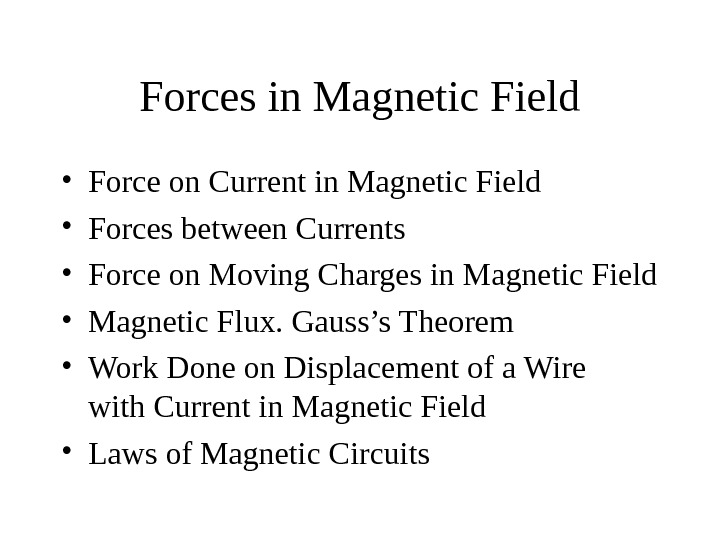Forces in Magnetic Field • Force on Current in Magnetic Field • Forces between Currents • Force on Moving Charges in Magnetic Field • Magnetic Flux. Gauss’s Theorem • Work Done on Displacement of a Wire with Current in Magnetic Field • Laws of Magnetic Circuits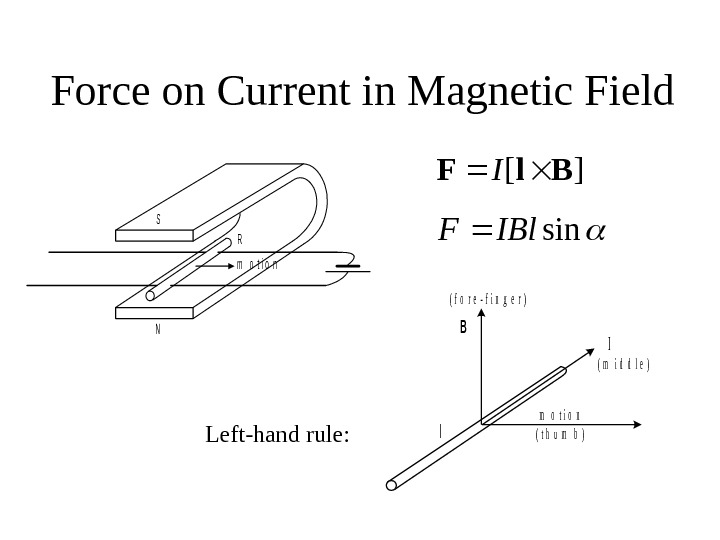Force on Current in Magnetic Fieldm o t i o n S N R B l I m o t i o n ( t h u m b ) ( m i d d l e ) ( f o r e — f i n g e r ) Left-hand rule: ][Bl. FI sin. IBl.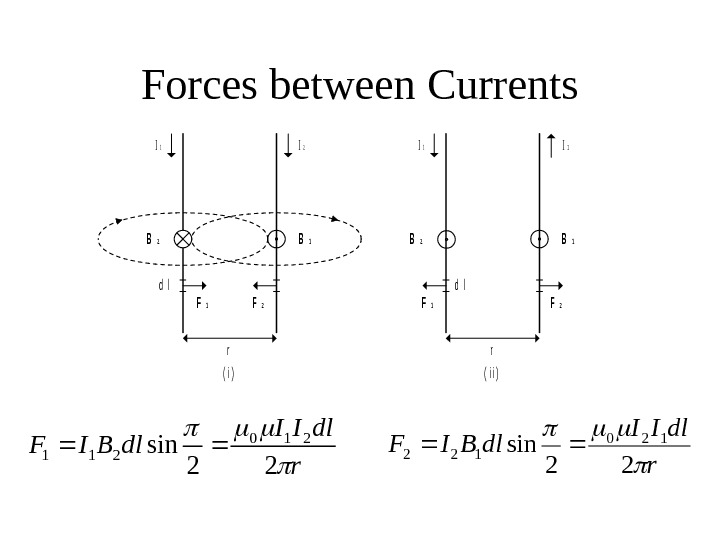Forces between Currentsr dl. II dl. BIF 22 sin 210 211 r dl. II dl. BIF 22 sin 120 122 I 1 I 2 B 2 B 1 d l F 1 F 2 rr ( i )( i i )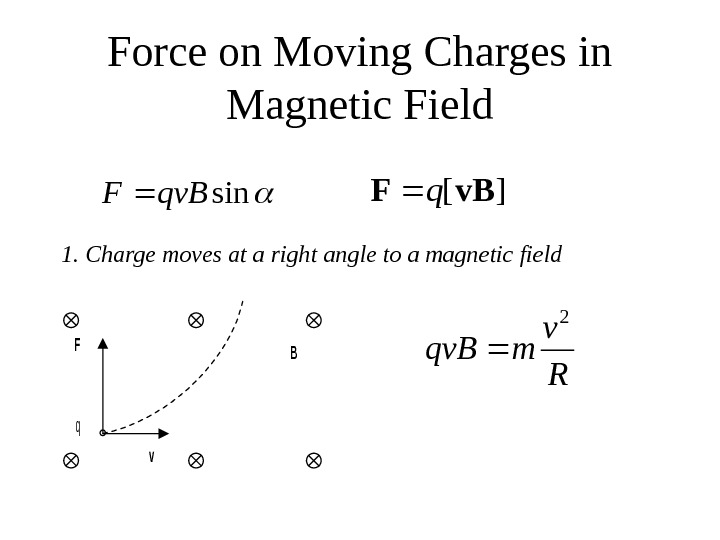Force on Moving Charges in Magnetic Fieldsinqv. BF][v. BFq 1. Charge moves at a right angle to a magnetic field F q v B R v mqv.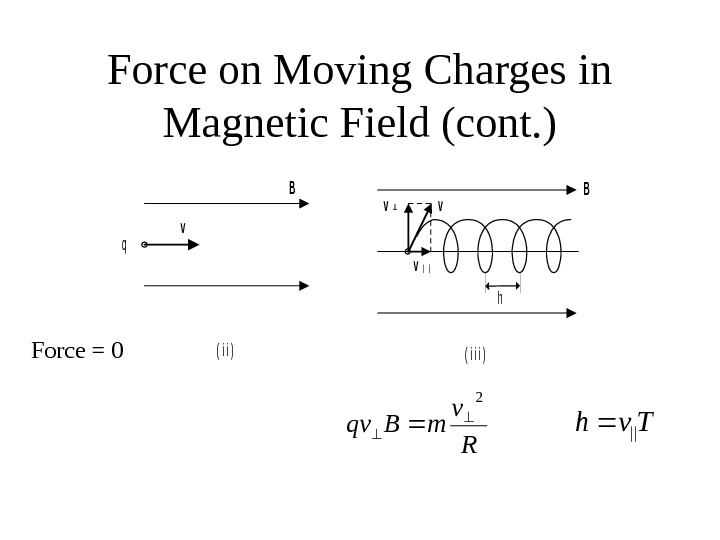Force on Moving Charges in Magnetic Field (cont. )B ( i i ) v q B vv v| | ( i i i ) h Force = 0 R v m. Bqv 2 Tvh||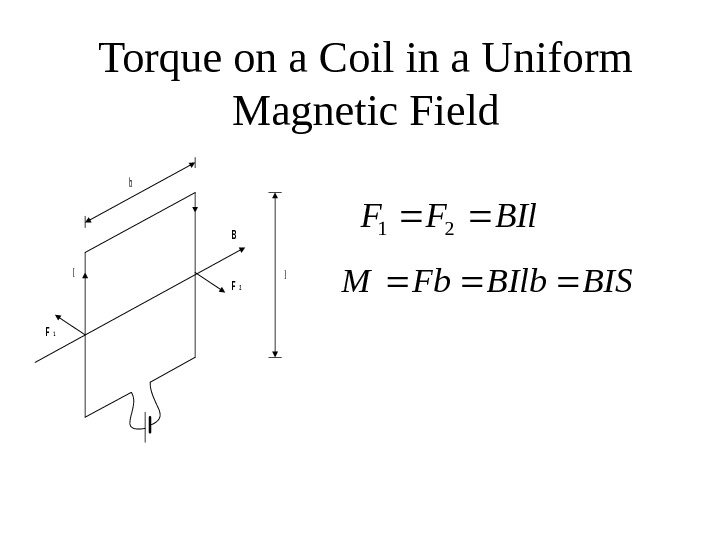Torque on a Coil in a Uniform Magnetic Fieldb l B F 2 F 1 I BIl. FF 21 BISBIlb. Fb. M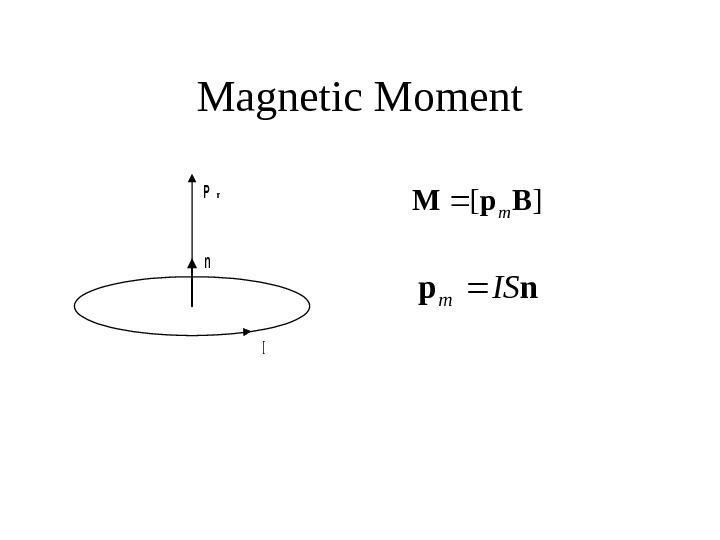Magnetic Moment. Pm n I ][ Bp. M m np IS m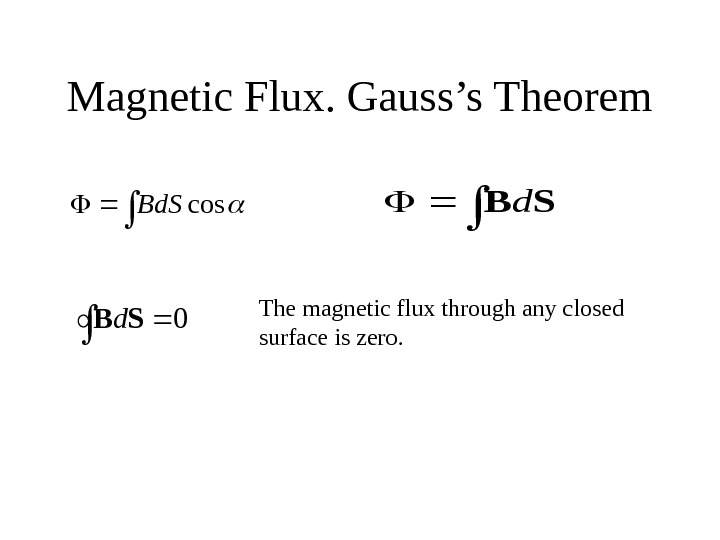Magnetic Flux. Gauss’s Theorem cos. Bd. S SBd 0 SBd The magnetic flux through any closed surface is zero.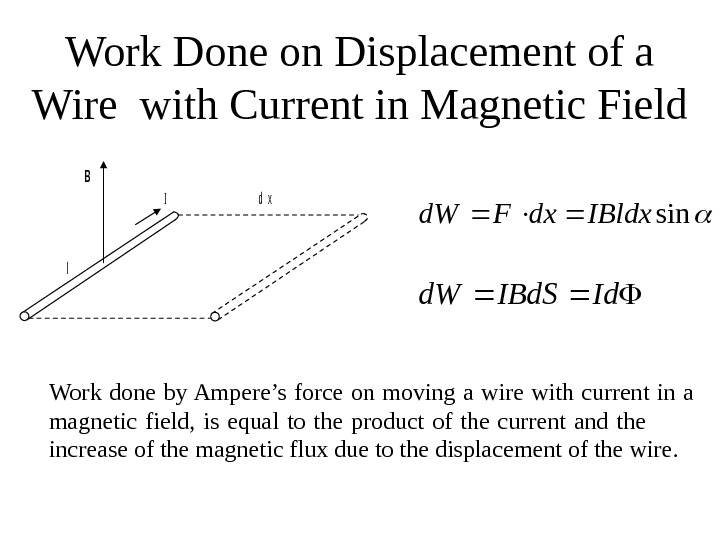Work Done on Displacement of a Wire with Current in Magnetic Field. B l Id x sin. IBldxdx. Fd. W Id. IBd. Sd. W Work done by Ampere’s force on moving a wire with current in a magnetic field, is equal to the product of the current and the increase of the magnetic flux due to the displacement of the wire.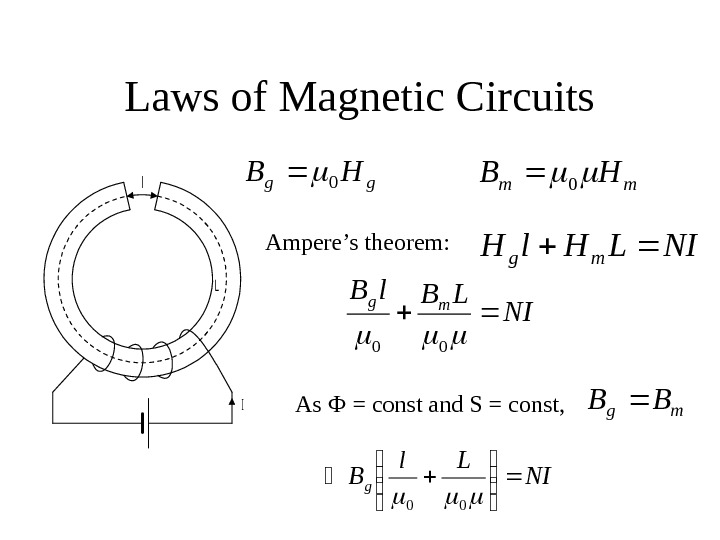Laws of Magnetic Circuits. L l I gg. HB 0 mm. HB 0 NILHl. Hmg NILBl. B mg 00 Ampere’s theorem: mg. BB NI Ll Bg 00 As Ф = const and S = const,Laws of Magnetic Circuits (cont. ) NI is called usually the magnetomotive force , MMF. S L S l NI SBg 00 S L S l 00 — is called the reluctance of the magnetic circuit.